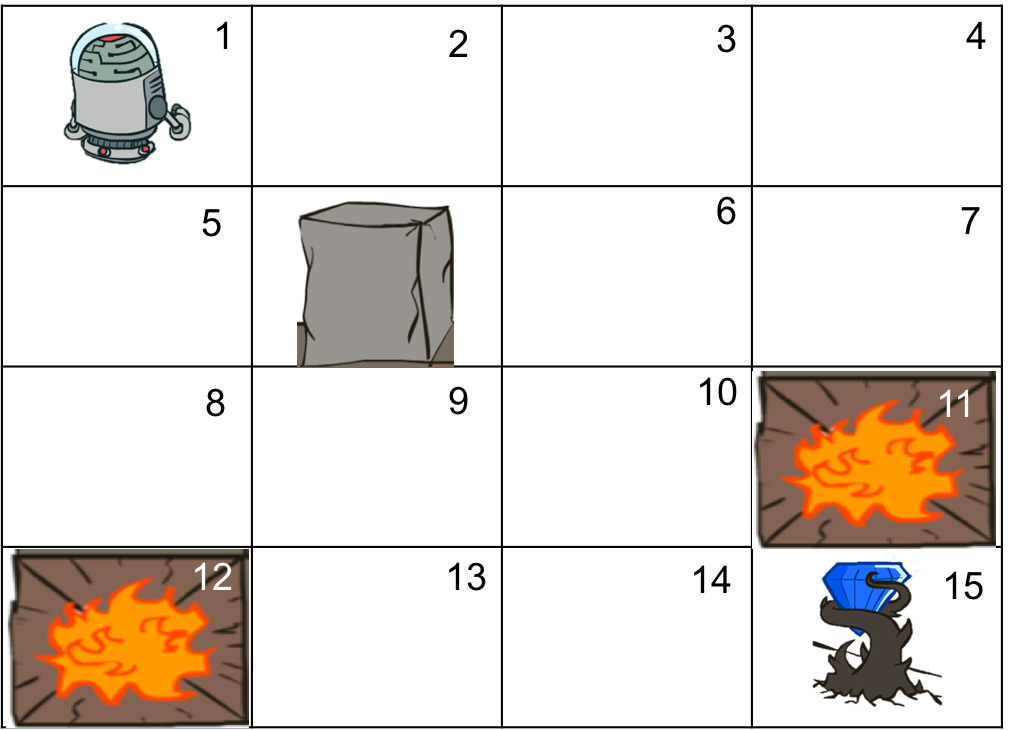## 关于

1. 环境未知情况下的更好的学习方法？
2. 蒙特卡罗方法和时间差分学习
3. SARSA, Q-learning
4. on-policy 与 off-policy 学习

1. 高中数学水平且年满18岁，部分内容需要你了解监督学习，和强化学习基本概念，你可以通过本教程前面的章节进行学习。
2. 如果你需要完成实践部分，需要有基本的 python 知识，你可以通过python快速入门快速了解python如何使用。

## 蒙特卡罗方法$$Q_n(s, a) = \frac{1}{n}\sum_{i=1}^n G_i$$

$$Q_n(s, a) = \frac{1}{n}(G_n + (n-1) Q_{n-1}(s, a)) \\ = Q_{n-1}(s, a) + \frac{1}{n}\left( G_n - Q_{n-1}(s, a) \right)$$